# Linear Applications of OP-AMP

## Current Controlled Current Source (CCCS) Circuit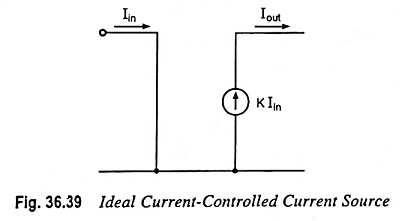Current Controlled Current Source (CCCS) Circuit: An ideal form of a Current Controlled Current Source (CCCS) Circuit by an input current is shown in Fig. 36.39. Such a circuit provides an output current dependent on the input current. A practical form of the circuit is built using an op-amp, as shown in Fig. 36.40. Output …

## Current Controlled Voltage Source (CCVS) Circuit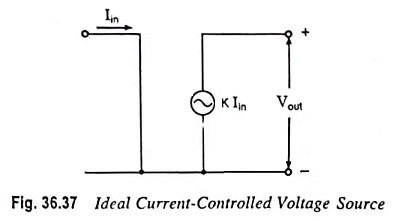Current Controlled Voltage Source (CCVS) Circuit: An ideal form of Current Controlled Voltage Source (CCVS) Circuit providing an output voltage controlled by an input current is shown in Fig. 36.37. The output voltage Vout is dependent on the input current Iin. A practical form of the circuit is built using an op-amp as shown in Fig. …

## Voltage Controlled Current Source (VCCS) Circuit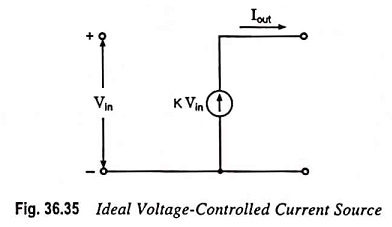Voltage Controlled Current Source (VCCS) Circuit: An ideal form of a current source, whose output current Iout is controlled by an input voltage is shown in Fig. 36.35. Here, the output current Iout is dependent on input voltage Vin. A practical circuit can be built, as shown in Fig. 36.36, with the output or load current …

## Voltage Controlled Voltage Source (VCVS) Circuit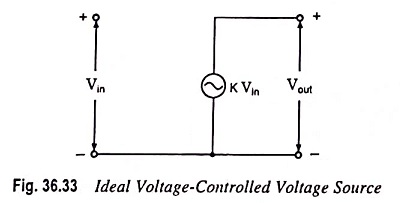Voltage Controlled Voltage Source (VCVS) Circuit: An ideal form of a voltage source, whose output voltage Vout is controlled by an input voltage Vin, is given in Fig. 36.33. The output voltage is seen to be dependent on the input voltage (times a scale factor K). Such a circuit can be built by connecting an …

## Current to Voltage Converter Circuit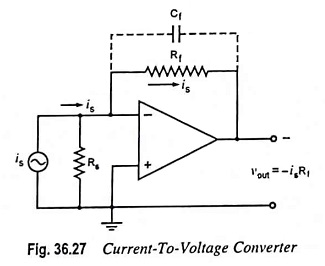Current to Voltage Converter Circuit: A device that produces a voltage proportional to input signal current is called a Current to Voltage Converter Circuit. The circuit arrangement is shown in Fig. 36.27. In this circuit, a photocell or photomultiplier tube that provides output current proportional to the light flux but independent of the load impedance, …

## Voltage to Current Converter CircuitVoltage to Current Converter Circuit: Occasionally in industrial electronics, it is necessary to provide a current proportional to certain voltage, even though the load impedance may vary. A circuit which can perform this job, is called a Voltage to Current Converter Circuit. The circuit of Voltage to Current Converter Circuit is shown in Fig. 36.25. …

## Summing, Scaling and Averaging Amplifier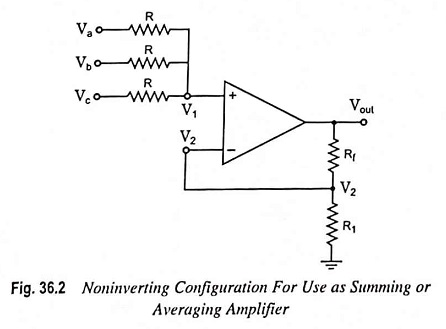Summing, Scaling and Averaging Amplifier: The most useful of the op-amp circuits employed in analog computers is the summing amplifier circuit. This circuit can be used to add ac or dc signals. This circuit provides an output voltage proportional to or equal to the algebraic sum of two or more input voltages each multiplied by …

Scroll to Top